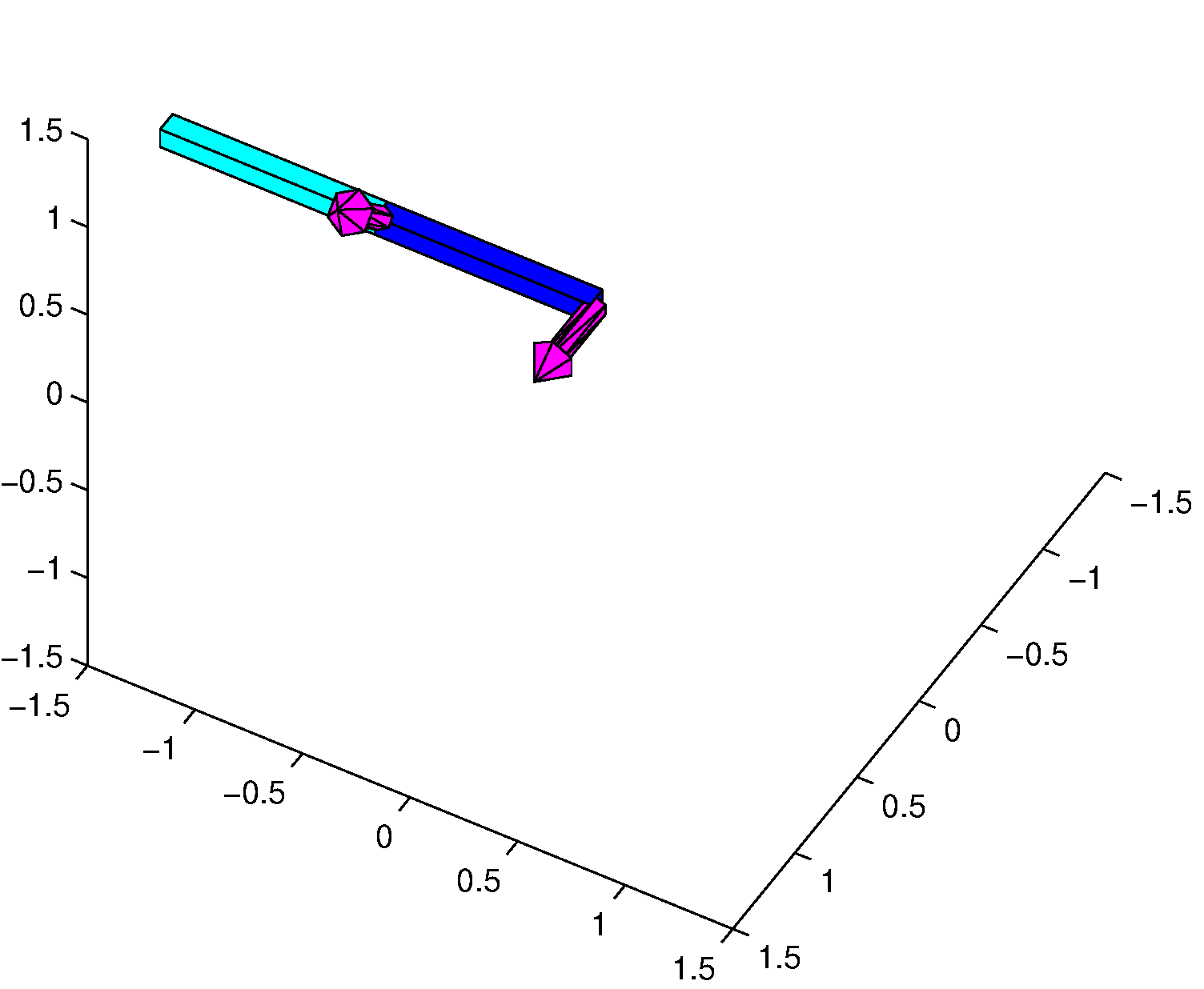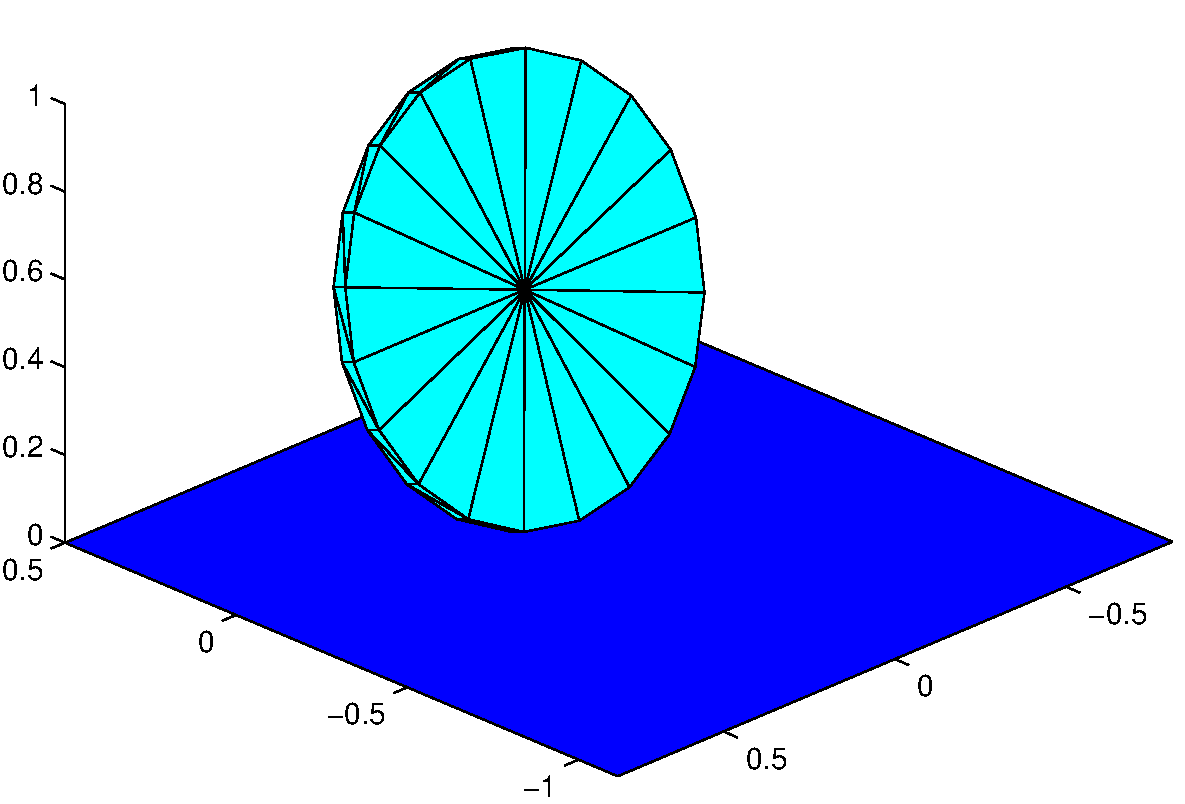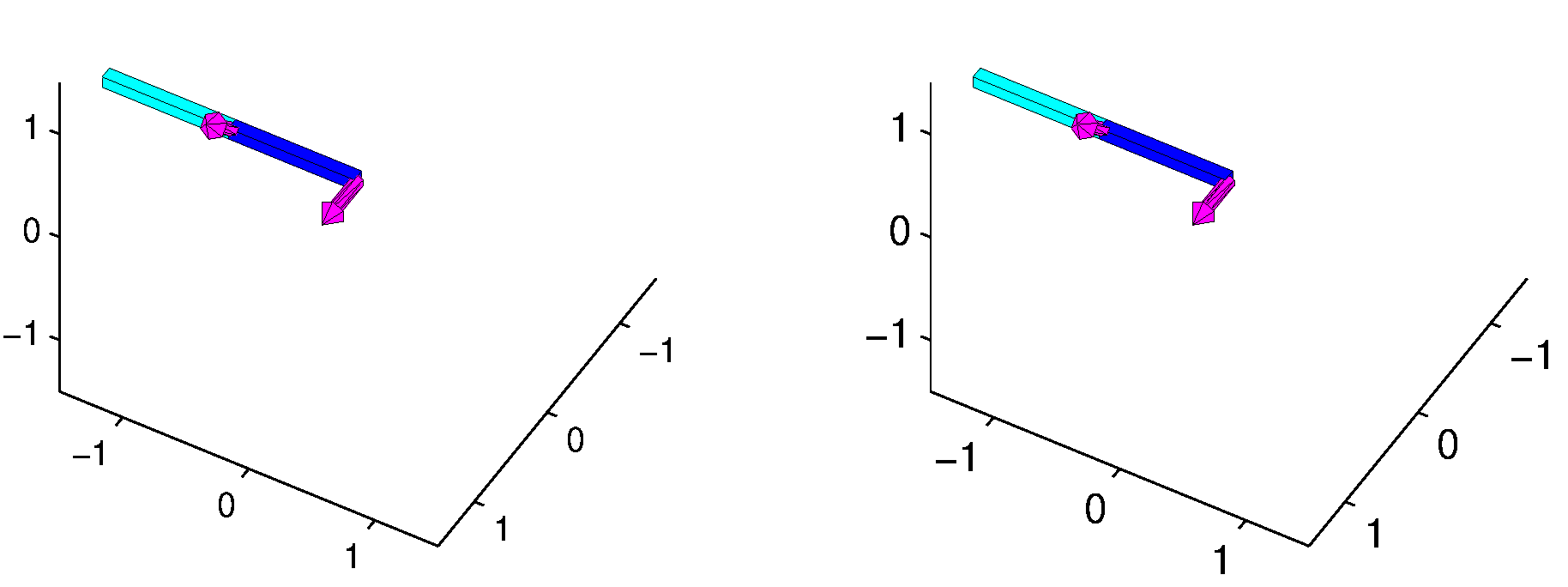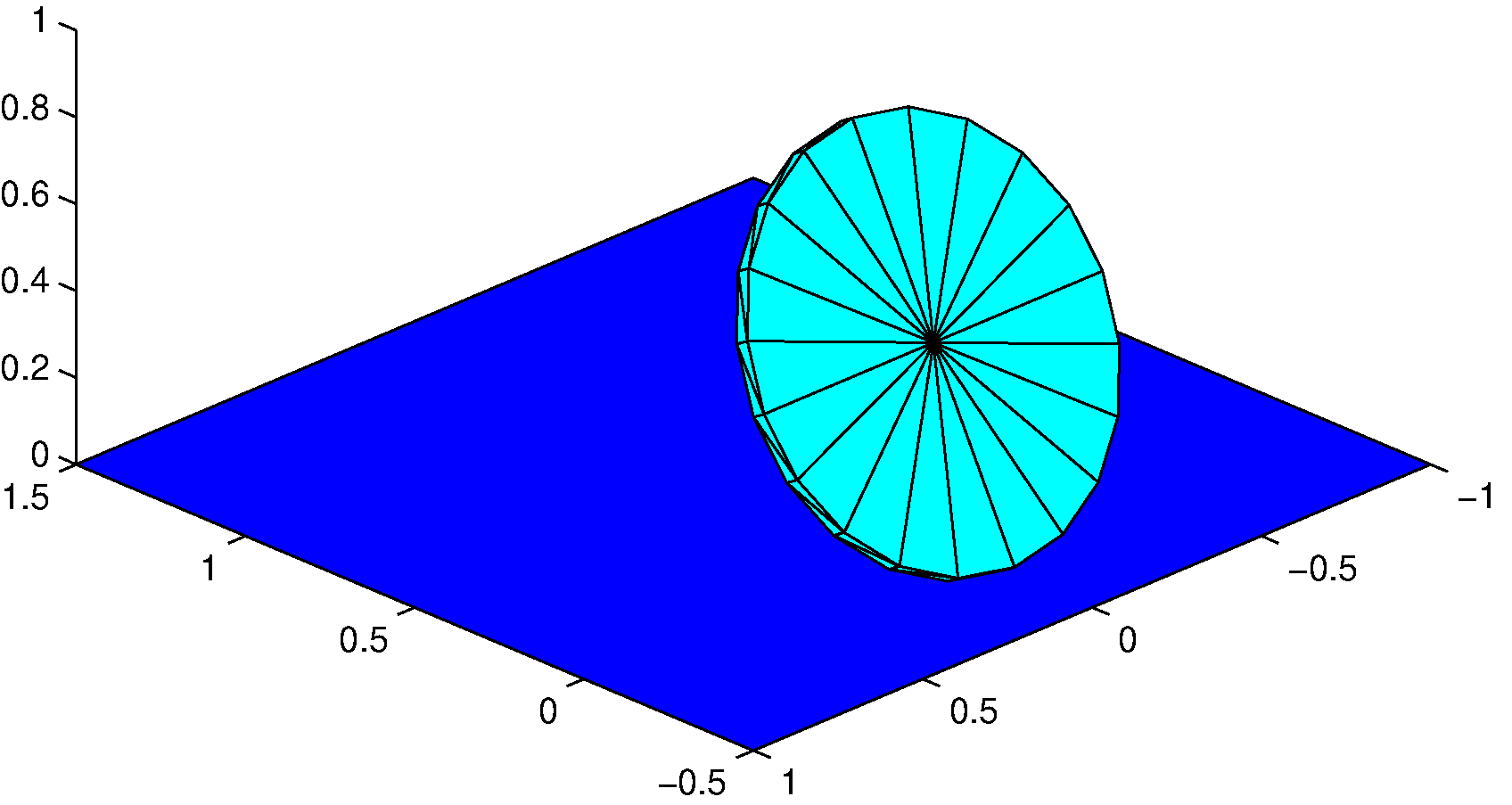top of page

#### 3D Dynamics Simulations

In spring of 2016 I competed the simulations on this page as the final project for MAE 6700 at Cornell University, taught by Professor Andy Ruina. The main focus of the course was to discuss 3D physical systems and analyze them using computer simulations in Matlab. For my final project I developed accurate simulations of a 3D double pendulum, and a 3D rolling disk/coin. On top of generating the physics simulations, animating the 3D results was also challenging. See Figure 1 for an animated example of the double pendulum simulation, and figure 2 for an example of the rolling disk simulation. This course is still taught, so I have avoided posting too many details about my code.

###### How I Approached this Problem

My general approach to this problem is the same that I used for the 2D animations discussed on my 2D Dynamics page. The overall process is to derive and manipulate the equations of motion using Matlab's symbolic toolbox (for simple systems this can be done by hand) into a form that can be handled by ode45. With a solution of this form we can run a simulation of the physical system using plausible initial conditions. See the 2D triple pendulum section for more details on how to actually implement this.Figure 1: 3D Double Pendulum Animation generated with ode45 in Matlab. Joint axes, shown in purple, are offset by 45 degrees. My solver allows arbitrary length links, with joint axes at any orientation. Note that small glitches appear in the animation where bodies overlap as a result of Matlab getting confused about what order to display patches in.Figure 2: 3D Rolling Disk Simulation generated in Matlab using ode45. My solver takes initial conditions and parameters, such as the mass of the disk, and solves for a given time span. The purple lines trace the motion of the center of the disk, and its path along the floor.

#### 3D Double Pendulum

The 3D double pendulum animations shown in this section (Figures 2 and 4) were generated in Matlab as a final project for MAE 6700, Advanced Dynamics, at Cornell University. This simulation is useful beyond a pure academics, because a sequential robot arm could be simulated using the same approach, with the simple addition of controllable torques about the hinge axes. Note that in all the animations shown here the joint axes are shown as purple unit vector arrows. These arrows are a visual aid only, and are not physical objects in the simulations.

3D Double Pendulum###### Angula Momentum Balance Solution

Figure 3: 3D Double Pendulum Simulation. The equations of motion where calculated in two different ways, using a Lagrangian approach, and a more traditional Newtonian angular momentum balance approach. Both methods produce identical results, within the limits of floating point precision. Note that small flickering glitches appear in the animation where bodies overlap as a result of Matlab getting confused about what order to display patches in. This simulation is identical to the one shown in Figure 1.

###### How I Approached this Problem:

The 3D double pendulum simulation shown here was computed using two different methods, a Lagrangian Approach and an angular momentum balance approach, to find the equations of motion. I then manipulate these equations into forms that are efficient for Matlab to solve using the differential equations solver "ode45." After specifying initial conditions and solving for a specified length of time, post processing includes calculating the potential and kinetic energy for each time step and generating a 3D animation. Both solutions use a minimal number of coordinates to describe the pendulum motion: θ1 and θ2 for the angle of each link with respect to its hinge. In this parameterization the first hinge is fixed to the global coordinate frame, and the second hinge is fixed to the frame of first link. This is essentially an axis-angle description, but in my code I use rotation matrices to describe the orientation of each body (converting from axis-angle to rotation matrix with symbolic variables). Rotation matrices were selected for ease of implementation in Matlab, despite their computational deficiencies, as opposed to a quaternion based system.

Because this project is still offered as a final project I have not posted any of the actual code, for details about how to approach this problem in Matlab, see my explanation of the 2D triple pendulum. The overall approach is essentially identical, except for the additional complication of 3D rotations.Figure 4: Double Pendulum Total Energy, corresponding to the simulation shown in Figure 2. The total system energy remains constant throughout the simulation, and is identical for both solution methods. Note that the total system energy is zero, because the pendulum starts with zero kinetic energy, and with the center of mass of both links start at z = 0.

###### Accuracy Verification

I used several different methods to confirm the dynamic accuracy of these simulations. First, I solved the system using two independent methods, and got identical results. Second, as shown in Figures 4 and 7, the total system energy remains constant throughout the duration of the simulation, as we should expect. Third, though an arbitrary double pendulum will probably be chaotic, it is possible to test the system's behavior against predictable subsets of solutions. For example, Figure 5 shows a system where the rotation axis of the second link is aligned with its center of mass. In this situation, the center of mass of the second link is locked with respect to the first link so that the overall system behaves link a simple single link pendulum, which exhibits completely periodic motion. To verify that the motion is periodic, look to Figures 6 and 7, which show the raw angles output by the solver, and the system energy. In this situation there is also no net torque acting on the second link, about its axis of rotation, so its orientation can be seen to remain constant throughout the animation. Finally, I was able to compare the simulation to those of other students, who had achieved the same results.

It is important to note that if the simulations are run for long enough we would expect the systems to lose accuracy due to roundoff error, but this is not a significant problem within the time spans considered here.Figure 5: Aligned Rotation Axis. In this simulation the second hinge axis is aligned with the center of mass of second link. In this situation the motion of the system is completely periodic, rather than chaotic, because the center of mass of the second link is locked with respect to the first link, and the system is essentially a simple pendulum. This configuration also has the effect of allowing the second link to perfectly maintain its orientation throughout the motion. Figures 5 and 6, showing the angles of each hinge and the system energy, respectively, confirm that the system is periodic.Figure 6: Periodic Rotation Angles, corresponding to the simulation shown in Figure 5. It is important to note that the rotation of the second link is measured with respect to a coordinate frame that is fixed to the first link, so even as the second link maintains orientation with respect to the global frame, it must rotate with respect to the first link.

Figure 7: Periodic System Energy, corresponding to the simulation shown in Figures 5 and 6. The system energy is perfectly periodic, matching up with the period shown in Figure 6. Note that the total system energy is positive, because the pendulum starts with zero speed with its center of mass above zero on the z-axis.

###### Notes on 3D Animations in Matlab

Generating 3D animations in Matlab can be challenging. I used patch objects to generate planar faces, and combine them to create 3D visuals. Unfortunately, Matlab does not handle patches overlapping very well, and the results can appear glitchy when patches overlap. The viewing perspective for the 3D axis should be set to make 3D motions easy to see. To make the results move I either iteratively generate each frame and save it to a GIF or movie file, or repeatedly plot it on the screen for immediate inspection. To improve performance, it is much faster to update the GIF position data, and redraw the frame, than it is to generate a new patch. When live plotting results, I achieve a smoother looking result, by keeping track of the elapsed time since the last fame was posted and interpolating the next frame, allowing for a variable frame rate.

3D Animations

#### Rolling Disk

This Matlab simulation is meant to emulate the motion of a coin or other disk rolling on a flat surface. Unlike the pendulum simulations, this is not a chaotic system, and generally produces predictable periodic or quasi-periodic results. Figure 7 shows a fairly typical motion for arbitrary initial conditions, with the disk moving in all three directions and rotating about every axis.

3D Rolling DiskFigure 7: Rolling Disk with Quasi-Periodic Motion. This simulation shows the disk rolling exhibiting a quasi-periodic motion, meaning that the motion is qualitatively periodic, but the motion pattern does not precisely line up with itself. If you observe closely, especially around the floor trace, you can see that the pattern does not quite line up as the disk moves through its second iteration. The purple floor and center trace shown in this image are a visual aid only, and are not physical bodies in the simulation. Note, for viewing purposes this animation is displayed at half speed.

###### Assumptions

This rolling disk simulation is complicated, but there are a few assumptions that we can make to ease computation. First, we assume that the disk is always rolling, meaning at the point where the disk touches the ground the disk is not moving relative to the ground. For this to be true in all situations we are essentially assuming that there is an unlimited friction force between the disk and the ground that maintains this constraint. Second, the disk is assumed to be two dimensional at the point where it contacts the ground, so that as the disk leans to one side or the other (and is not rolling forward), the contact point between the disk and the ground does not change. In the animations the thickness of the disk is a visual cue that is not actually factored into the simulation. Also note that the simulation does not need to account for possibly of the coin hitting the ground.

###### Conventios

With these assumptions we can use a minimal parameterization that describes the motion of the disk with only three variables. Because disk cannot side along the ground, only roll, it is possible to completely define the motion of the disk, from one moment to the next, in terms of the 3D angles the disk makes with the ground. That is, given exactly knowledge of the starting point, and how the disk rotates in 3D space, it is possible to determine its 3D Position at any time. Note, however, that this calculation is cumulative, meaning you need to solve for the x,y, z-position for each time step consecutively. The nature of this problem lends itself to being easy to define using Euler angles. Using the aircraft Euler angle convention (rotation about the global z, then the new y, and finally new x-axis), works well in this case. Gimbal lock is not a problem, because the disk tends to stay within a limited range of angles. For instance, the disk needs to lie completely on its side for the final x-axis to be aligned with the original z-axis, in which case the simulation is invalidated anyway. See Figure 8 for more details about how this convention is applied.Figure 8: The Rotations Convention for the rolling disk is identical to the Euler angle convention used to describe aircraft, however, it is best to avoid use of the terms "roll," "pitch," and "yaw," because this can cause confusion with what we generally would call the "rolling" of a coin or wheel. Instead, in this explanation, I use Φ (phi), θ (theta), ψ (psi), which are defines above. First the coordinate frame is rotated by Φ about k (z-axis). Second the frame is rotated by θ about the j' vector, which is the new y-axis. Last, the the frame is rotated by ψ about the i'' vector, which is the final x-axis created in the previous rotation. Note that the first frame is represented by vectors i,j,k, the second by i',j',k', and the third by i'',j'',k''.

###### Applying the Euler Angle Convention

Using the system described in Figure 8, we can now approach the system in the same way that I handled the 2D and 3D pendulum simulations. I have avoided posting my actual code, because this assignment is still used as a final project, but I have described the general outline. It is more difficult to apply the Lagrange approach in this system, because this system contains a non-holonomic constraint (that the velocity of the disk at the contact point is zero relative to the ground plane). For this reason I completed this problem using only the angular momentum balance approach. The best place to apply the angular momentum balance is at the contact point between the ground and the disk, so that reaction forces can be ignored. In my code I used rotation matrices to write out the angular momentum balance, so it is necessary to symbolically write the rotation matrix in terms of Φ, θ, ψ. All necessary vectors, matrices, velocities and accelerations can be defined in terms of Φ, θ, ψ, and their first and second derivatives, as defined in Figure 8, so a "right hand side" file can be set up in Matlab (using the symbolic toolbox) to let ode45 solve for these values for any length of time.

###### Finding X, Y, Z

While the system can be simulated using only Φ, θ, ψ, and their derivatives, we still need to calculate the x, y, and z position of the disk in order to display the animation properly. The z coordinate can easily be calculated as a function of θ ( z = r*cos(θ), where r is the radius of the disk), but the x and y coordinates are more challenging. Unlike the pendulum simulations, the relation between the rotations and the rotations and the x, y-position is a velocity constraint, rather than a kinematic constraint. The velocity of the center of mass of the disk can be calculated as follows:Where v is the vector velocity of the center of mass, ω is the angular velocity of the disk, and r is the vector from the contact point to the center of mass. Both r and ω can be written in terms of Φ, θ, ψ and their derivatives, so the velocity of the center of mass can also be calculated using these values. We can use ode45 (or any other ode solver) to get x and y for each time step by specifying a valid initial position and using the above relation. This can either be done after Φ, θ, ψ are solved, as part of the post processing, or at the same time, by adding this relation into the right hand side file generated to solve Φ, θ, ψ.

###### Animating the Results

Once we have values for Φ, θ, ψ, x, y, and z, it is relatively easy to animate the results using the same process used for all my other dynamics simulations: iteratively generating frames using patches to create 3D objects. The disk shown in my animations is built out of triangular patches (like an stl file), the vertices of which can be calculated for each frame by applying the rotations and position data. In this case the spokes formed by the edges of patches help make the rotation of the disk more visible. To make the motions easier to track over time, so that patterns can be observed, I added a purple traces to the animation to show the path of the center of mass and the contact point with the floor. I also added a thin thickness to the disk to make the motions easier to follow, though this is not addressed in the simulation.Figure 9: Slowly Rolling Disk. In this simulation we can see the disk lean over very far and then pop back up instead of falling over as you might expect if an actual coin was rolling in this way. This unusual behavior occurs because the simulation assumes that there is always a rolling constraint between the disk and the floor. This means that we are assuming that there is always a high enough friction force to enforce this condition, which is not necessarily realistic. It is important to note, however, that this is not an error in the simulation, merely a limitation based on assumptions.

###### Accuracy Verification

Although the solutions generated with the rolling disk simulation are not chaotic, they can produce results that look non-intuitive, such as those shown in Figure 9 where the disk seems to almost fall completely over and then pops back up. In this case the unusual motions are a result of the rolling constraint. A real coin would probably slide and fall flat on its side at the extreme lean angles shown, but the simulation assumes that there is enough friction to maintain the roiling condition. The presence of unusual results makes it important to verify the accuracy of the simulation numerically. The first way to do this is to check that the solutions have constant energy. Looking to Figure 10, which corresponds to the animation shown in Figure 9, we can see that the total system energy does indeed remain constant.Figure 10: Simulation Parameters corresponding to the animation shown in Figure 9. The energy calculation at the bottom shows that the total system energy remains constant for the entire simulation.

The second way to double check the accuracy of the system is to attempt to derive conditions that will allow for predictable solution sets. For instance, a disk that starts rolling in a perfectly straight line with no tilt should continue to do so indefinitely. A more complex derivation can be used to generate initial conditions that will result in perfectly periodic results, where the disk rolls in a perfect circle. This can be derived by assuming that θ is constant and the derivatives of Φ and ψ are constant (more specifically that their derivatives are zero). We can then specify either θ (the lean), or the derivative of Φ, or ψ (turning speed and rolling speed respectively), plug these values into the angular momentum balance, and use the system of 3 scalar equations (1 vector equation) to solve for the parameters left unknown. Looking to Figure 11, we can see that this derivation does produce circular rolling results. It is very difficult to generate perfectly circular motions by trial and error. Together with the energy calculations described above, I take this as proof that the simulation is accurate.Figure 11: Rolling Disk with Circular Motion. This simulation starts with very special initial conditions that balance the angular motion of the disk so that the motion is perfectly circular. Ignoring the eventual numeric breakdown of the simulation, the disk will circle indefinitely.

bottom of page